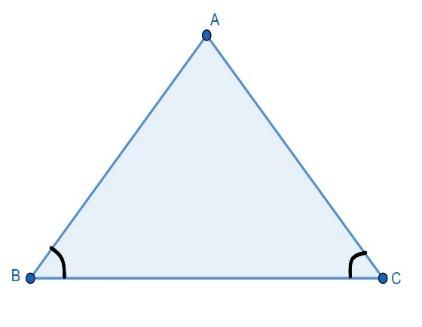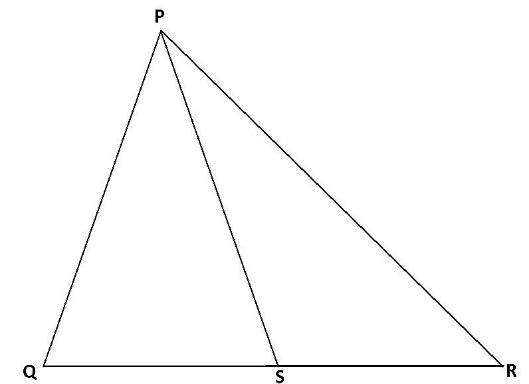QUESTION

# In a $\Delta PQR$ , $PS$ is the angle bisector of $\angle P$ and $\angle Q={{70}^{0}}$ , $\angle R={{30}^{0}}$, then,(a) $QS > PQ > PR$(b) $QS < PQ < PR$(c) $QS < PQ > PR$(d) None of these

Hint: For solving this question we will use three important results of a triangle like the sum of internal angles of the triangle, properties of an isosceles triangle and how to apply sin rule in any triangle. Then we will solve to find the correct answer.

Given:
A $\Delta PQR$ in which $PS$ is the angle bisector of $\angle P$ and $\angle Q={{70}^{0}}$ , $\angle R={{30}^{0}}$ .
Now, we will see 3 important results related to triangles one by one which will be used to solve this problem.In $\Delta ABC$ following points should be considered:
1. Sum of interior angles of a triangle is ${{180}^{0}}$ . So, $\angle A+\angle B+\angle C={{180}^{0}}$ .
2. If Angles opposite to equal sides of an isosceles triangle are equal. So, if $\angle B=\angle C$ then, $AB=AC$ . The reverse of this is also true.
3. According to sine rule: $\dfrac{BC}{\sin A}=\dfrac{AB}{\sin C}=\dfrac{AC}{\sin B}$ .
Now, we come back to our question. We have $\Delta PQR$ as shown in the figure below:It is given that, $\angle Q={{70}^{0}}$ and $\angle R={{30}^{0}}$ . Then, from the first property, we can write that, \begin{align} & \angle P+\angle Q+\angle R={{180}^{0}} \\ & \Rightarrow \angle P+{{70}^{0}}+{{30}^{0}}={{180}^{0}} \\ & \Rightarrow \angle P={{80}^{0}} \\ \end{align}
Now, as PS is the angle bisector of $\angle P$ . Then, $\angle QPS=\angle RPS={{40}^{0}}$ .
Now, consider $\Delta PQS$ . As $\angle PQS={{70}^{0}}$ and $\angle QPS={{40}^{0}}$ . Then, from the first property, we can write that,
\begin{align} & \angle PQS+\angle QPS+\angle PSQ={{180}^{0}} \\ & \Rightarrow {{70}^{0}}+{{40}^{0}}+\angle PSQ={{180}^{0}} \\ & \Rightarrow \angle PSQ={{70}^{0}} \\ \end{align}
Now, in $\Delta PQS$ as, $\angle PSQ=\angle PQS={{70}^{0}}$ . Then, from the second property, we can write that,
$PQ=PS................\left( 1 \right)$
Now, in $\Delta PQS$ we apply sine rule. Then,
\begin{align} & \dfrac{QS}{\sin \left( \angle QPS \right)}=\dfrac{PQ}{\sin \left( \angle PSQ \right)} \\ & \Rightarrow \dfrac{QS}{\sin {{40}^{0}}}=\dfrac{PQ}{\sin {{70}^{0}}} \\ & \Rightarrow \dfrac{QS}{PQ}=\dfrac{\sin {{40}^{0}}}{\sin {{70}^{0}}} \\ \end{align}
Now, as $\sin x$ increases in $x\in \left[ 0,{{90}^{0}} \right]$ . Then, $\sin {{40}^{0}}<\sin {{70}^{0}}$ . From this, we can write,
\begin{align} & \dfrac{QS}{PQ}=\dfrac{\sin {{40}^{0}}}{\sin {{70}^{0}}} \\ & \Rightarrow \dfrac{QS}{PQ} < 1 \\ & \Rightarrow QS < PQ...........\left( 2 \right) \\ \end{align}
Now, in the $\Delta PSR$ . As $\angle RPS={{40}^{0}}$ and $\angle PRS={{30}^{0}}$applying first property. Then,
\begin{align} & \angle PSR+\angle RPS+\angle PRS={{180}^{0}} \\ & \Rightarrow \angle PSR+{{40}^{0}}+{{30}^{0}}={{180}^{0}} \\ & \Rightarrow \angle PSR={{110}^{0}} \\ \end{align}
Now, apply sine rule in $\Delta PSR$ . Then,
\begin{align} & \dfrac{PS}{\sin \left( \angle PRS \right)}=\dfrac{PR}{\sin \left( \angle PSR \right)} \\ & \Rightarrow \dfrac{PS}{\sin \left( {{30}^{0}} \right)}=\dfrac{PR}{\sin \left( {{110}^{0}} \right)} \\ & \Rightarrow \dfrac{PS}{PR}=\dfrac{\sin {{30}^{0}}}{\sin \left( {{180}^{0}}-{{70}^{0}} \right)} \\ & \Rightarrow \dfrac{PS}{PR}=\dfrac{\sin {{30}^{0}}}{\sin {{70}^{0}}} \\ \end{align}
Now, as $\sin x$ increases in $x\in \left[ 0,{{90}^{0}} \right]$ . Then, $\sin {{30}^{0}}<\sin {{70}^{0}}$ . From this, we can write,
\begin{align} & \dfrac{PS}{PR}=\dfrac{\sin {{30}^{0}}}{\sin {{70}^{0}}} \\ & \Rightarrow \dfrac{PS}{PR}<1 \\ & \Rightarrow PR>PS \\ \end{align}
Now, from equation (1) we get:
\begin{align} & PR>PS \\ & \Rightarrow PR>PQ.............\left( 3 \right) \\ \end{align}
Now, from equation (2) and (3) we get:
$QS < PQ < PR$
Hence, (b) is the correct option.

Note: Here, the student must apply the properties of triangles in a correct way and application of sine rule should be used only to relate sides of the same triangle not any side of other triangles. Moreover, students should avoid any error related to calculation while writing inequalities symbols.